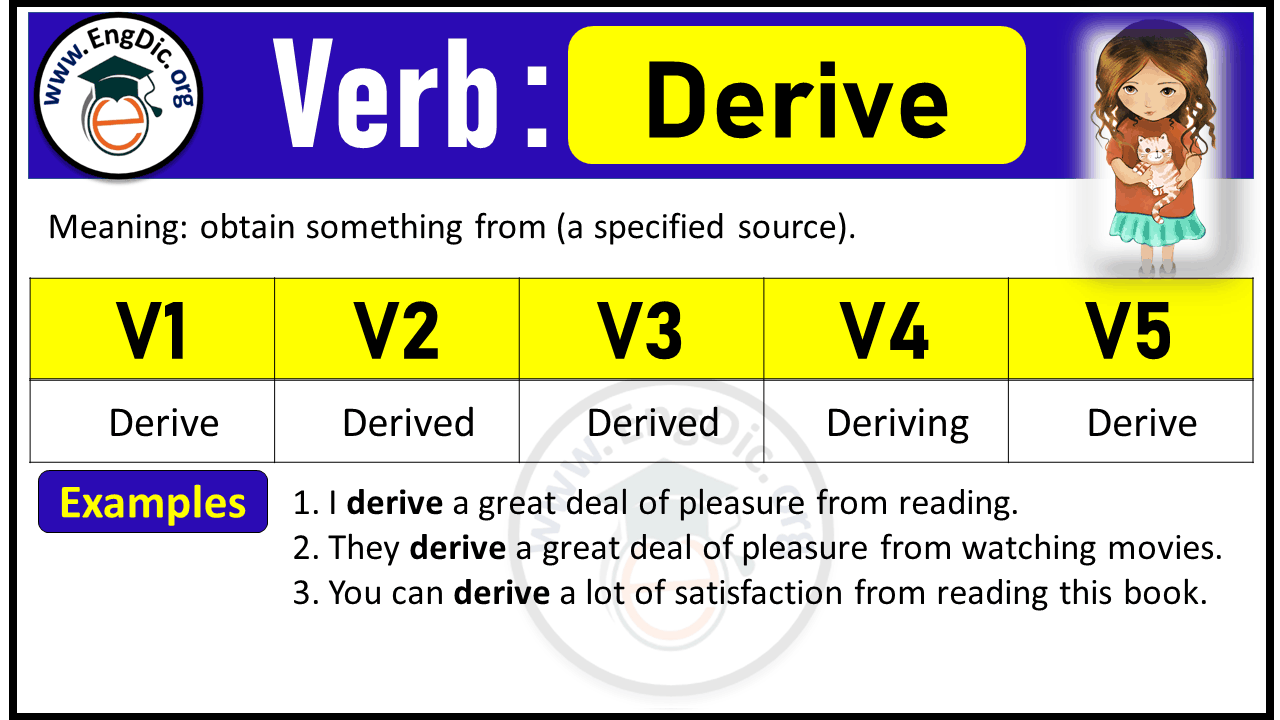# Derive Verb Forms: Past Tense and Past Participle (V1 V2 V3)

Meaning: obtain something from (a specified source).

## Derive Verb Forms V1 V2 V3 V4 V5

 Infinitive/Base Form (V1): Derive Past Tense (V2): Derived Past Participle Form (V3): Derived Present Participle/Gerund (V4): Deriving 3rd Person Singular (V5): Derives

## Derive Past Tense:

Past Tense of Derive is Derived.

Example: Sarah derived the quadratic equation.

## Derive Past Participle:

Past Participle Form of Derive is Derived.

Example: Sarah has derived the quadratic equation.

## Derive Present Participle:

Present Participle Form of Derive is Deriving.

Example: Sarah is deriving the quadratic equation.

## Derive 3rd Person Singular:

3rd Person Singular of Derive is Derives.

Example: Sarah derives the quadratic equation.## Derive Conjugation

### Indefinite / Simple Present Tense

• I derive the quadratic equation.
• We/You/They derive the quadratic equation.

### Present Continuous Tense

• I am deriving the quadratic equation.
• We/You/They are deriving the quadratic equation.

### Present Perfect Tense

• I have derived the quadratic equation.
• We/You/They have derived the quadratic equation.

### Present Perfect Continuous Tense

• I have been deriving the quadratic equation.
• We/You/They have been deriving the quadratic equation.

### Indefinite / Simple Past Tense

• I derived the quadratic equation.
• We/You/They derived the quadratic equation.

### Past Continuous Tense

• I was deriving the quadratic equation.
• We/You/They were deriving the quadratic equation.

### Indefinite / Simple Future Tense

• I will derive the quadratic equation.
• We/You/They will derive the quadratic equation.

### Future Continuous Tense

• I will be deriving the quadratic equation.
• We/You/They will be deriving the quadratic equation.

### Future Perfect Tense

• I will have derived the quadratic equation.
• We/You/They will have derived the quadratic equation.

### Future Perfect Continuous Tense

• I will have been deriving the quadratic equation.
• We/You/They will have been deriving the quadratic equation.

## Past Tense of Derive Phrasal Verbs

 Derive Phrasal Verbs Past Tense Derive from Derived from Derive off Derived off Derive out Derived out Derive over Derived over Derive through Derived through Derive up Derived up Derive with Derived with Derive in Derived in Derive at Derived at Derive toward Derived toward

## FAQS

### What is the Future Tense of Derive?

Future Tense of derive is “will derive”.

### What is the Present Tense of Derive?

Present Tense of derive is “derive + s/es or ing”.

 I/we/you/they derive He/she/it/singular name derives Present participle deriving

### What is the Past Perfect Tense of Derive?

Past perfect tense of take is “had derived”.

Explore Other Verb Forms:

Last updated on May 21st, 2023 at 09:50 am